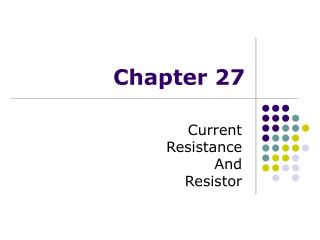# Chapter 27 - PowerPoint PPT PresentationDownload PresentationChapter 27

Chapter 27Download Presentation## Chapter 27

- - - - - - - - - - - - - - - - - - - - - - - - - - - E N D - - - - - - - - - - - - - - - - - - - - - - - - - - -
##### Presentation Transcript

1. Chapter 27 Current Resistance And Resistor

2. Review The current is defined and its unit is ampere (A), a base unit in the SI system. + + A + + + + I The charges passing through the area could be positive or negative or both. They are called charge carriers. Current direction is chosen to the electric field direction or the direction as the flow of positive charges.

3. Review The motion of the charge carriers is the result of the movement driven by the electric field plus random collisions with other particles in the conductor. The average velocity of this motion is called the drift velocity The current and the drift velocity has a linear relationship. E n is the number of charge carriers per unit volume. q is the carrier charge The drift velocity is very small. What is actually prorogating in the conducting wire is the electric field. So the conducting wire is actually the carrier or guide for the electric field, which delivers the electric power.

4. Current Density J, the definition The current densityJ of the current I a conductor is defined as the current per unit area Current density is a vector and is in the direction of the positive charge carriers, or in the direction of the electric field. Compare with, one has: J has SI units of A/m2

5. For some materials, the current density is directly proportional to the electric field: Conductivity and Ohm’s Law The constant of proportionality, σ, is called the conductivity of the conductor. Another often used parameter is resistivity, ρ, is just inverse of the conductivity: ρ = 1/σ And this formula, is called Ohm’s Law.

6. Ohm’s Law • Ohm’s law states the ratio of the current density to the electric field is a constant σ • Mathematically, J = σ E • Most metals obey Ohm’s law • Materials that obey Ohm’s law are said to be ohmic But • Not all materials follow Ohm’s law • Materials that do not obey Ohm’s law are said to be nonohmic • Ohm’s law is not a fundamental law of nature • Ohm’s law is an empirical relationship valid only for certain materials

7. From Ohm’s Law: Resistance and a more popular form of Ohm’s Law One has: Now exam the section of wire with length and cross section A, one has: Now we define a new parameter, the resistance of this section of the wire, R: Ohm’s Law becomes or

8. Resistance, discussion • SI units of resistance are ohms (Ω) • 1 Ω = 1 V / A • SI units of resistivity are Ω·m • Resistance proportional to the resistivity constant of the material, the length of the conductor (wire), inversely proportional to the cross section. • In a conductor, the voltage applied across the ends of the conductor is proportional to the current through the conductor • The constant of proportionality is called the resistance of the conductor

9. Resistivity Values and the temperature coefficient α

10. Resistance and Resistors All (almost) materials have resistance Those that are call ohmic if Ohm’s Law holds. A device made to have certain resistance value is call a resistor.

11. Ohmic Material, Graph • For an ohmic device • The resistance is constant over a wide range of voltages • The relationship between current and voltage is linear • The slope is related to the resistance

12. Nonohmic Material, Graph • Nonohmic materials are those whose resistance changes with voltage or current • The current-voltage relationship is nonlinear

13. Resistance and Temperature • Over a limited temperature range, the resistivity of a conductor varies approximately linearly with the temperature • ρo is the resistivity at some reference temperature To • To is usually taken to be 20oC • αis the temperature coefficient of resistivity • SI units of αare oC-1 • With a resistor: • This is often used to measure temperature with materials that have large α

14. Resistivity and Temperature, Graphical View • For some metals, the resistivity is nearly proportional to the temperature • A nonlinear region always exists at very low temperatures • The resistivity usually reaches some finite value as the temperature approaches absolute zero

15. Electrical Power: work down by the electric field in the circuit • As a charge Q moves from a to b, the electric potential energy of the system increases by QDV • This energy comes from the chemical energy in the battery • As the charge moves through the resistor (c to d), the system loses this electric potential energy, turning it to heat by the resistor • The electric power (energy time rate) the resistor consumes is Q Q because so With Ohm’s Law:

16. Electric Power, to summarize • The electric power is given by the equation: and is always correct. • If Ohm’s Law is applicable, alternative expressions are often useful: • Units: I is in A, R is in Ω, V is in V, and is in W

17. Example: Electric Power Transmission The question: Dallas needs 100 MW electric power which is generated 100 miles away. The transmission line (aluminum) has a diameter of 2 inches. How much power must be generated to deliver 100 MW to Dallas, (a) If 110 V is used? (b) if 600,000 V is used?